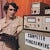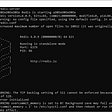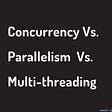# Encoding a whole computer in a type in Idris

Two things I learned from this:

1. If you write objects with values encoded at both runtime and compile time, you can use type classes to guide Idris through induction on them rather than having to reimplement it as proof code. Not only that, but the compiler can act as a better proof assistant by letting you know exactly what implementations are required and which ones are missing. That helped a lot.
2. Encoding data in type level lists that can mutate is hard, but it’s actually not the hardest thing I’ve tried to do in idris. I’ll describe at least one process for this.

## Guiding Idris through induction using interfaces

So I’ve often run into hassles showing Idris that something like (a > b) => (a - b) > 0, and these grow when the complexity of the operations increases. Taking your code, decomposing what it does in proof code, then reassembling the result in the form of proof is a drag after the fact. What you need to do is have values that encode their value in their type, but working on those can be hard as well. You don’t know what to call their types and how to identify the type of things can get out of hand.

If you’ve tried naively to prove something that uses the Integer relational operators, you’ve likely run into idris not really having a lot of insight. Proof code can’t look inside these even when the values are statically known since the relational operators are provided by interfaces that are fulfilled by builtin functions (and idris doesn’t have any insight into these). You can try to complete these proofs by using LTE, but I find that I’m kind of not smart enough. I need more help than idris is capable of providing (i used to get by with the tactic based prover, but even then wrote absurdly dumb proof code) but there really is a better way.

Consider this type:

`data SignedNat : Bool -> Nat -> Type where  Neg : (n:Nat) -> SignedNat True n  Pos : (n:Nat) -> SignedNat False n`

It both contains a runtime representation of the value and encodes the corresponding value in its type. If you hold this then idris at least conceptually knows what its value is, so that’s one thing: you don’t need to worry about how you’ll figure out what it contains because you’ll be required at each level to keep track (it’s a magic proof assistant).

Now you might look at this and think: It’s just more a more complicated Nat! How does this help us with proofs?

So the general strategy is that any operation we want to provide here, we’ll do so with symbols in our idris code. This will allow this number representation to give us analytic power we haven’t had before:

`public exportinterface GreaterSN sna snb where  gtrSN : sna -> snb -> Bool`

It doesn’t look like much but the types here carry helpful info. As long as a ?postpone isn’t used, idris has access to the implementation that goes along with a specific value and therefore any necessary induction via “auto variables” that declare interfaces needed:

`public exportimplementation (GreaterSN (SignedNat False a) (SignedNat False b)) => GreaterSN (SignedNat False (S a)) (SignedNat False (S b)) where  gtrSN (Pos (S a)) (Pos (S b)) = gtrSN (Pos a) (Pos b)`

This takes “GreaterSN (SignedNat False a) (SignedNat False b)” and provides “GreaterSN (SignedNat False (S a)) (SignedNat False (S b))”, but it does some nice things. You can write this without specifying what implementations are required and idris will tell you if they’re missing (or if you specify them here and they can’t be fulfilled). So it’s very assisitant-y. Importantly, idris doesn’t need any help to know the outcome when these have nice base cases like:

`public exportimplementation GreaterSN (SignedNat False Z) (SignedNat False _) where  gtrSN _ _ = False`

This works (you might be breathing a sigh of relief if you’ve tried proving things about numbers naively):

`proveNeg15GreaterThanNeg30 : gtrSN (Neg 15) (Neg 30) = TrueproveNeg15GreaterThanNeg30 = Refl`

So you can provide even fairly complicated things this way and they’re 100% understood by idris:

`public exportinterface SubSN sna snb where  subSN : sna -> snb -> (Bool, Nat)public exportimplementation SubSN (SignedNat _ _) (SignedNat _ Z) where  subSN (Neg x) _ = (True, x)  subSN (Pos x) _ = (False, x)public exportimplementation SubSN (SignedNat True _) (SignedNat False _) where  subSN (Neg x) (Pos y) = (True, x + y)public exportimplementation SubSN (SignedNat False _) (SignedNat True _) where  subSN (Pos x) (Neg y) = (False, x + y)public exportimplementation SubSN (SignedNat False Z) (SignedNat False (S n)) where  subSN (Pos Z) (Pos (S n)) = subSN (Neg 1) (Pos n)public exportimplementation SubSN (SignedNat True Z) (SignedNat False (S n)) where  subSN (Neg Z) (Pos (S n)) = subSN (Neg 1) (Pos n)public exportimplementation SubSN (SignedNat False n) (SignedNat False m) => SubSN (SignedNat False (S n)) (SignedNat False (S m)) where  subSN (Pos (S n)) (Pos (S m)) = subSN (Pos n) (Pos m)public exportimplementation SubSN (SignedNat True n) (SignedNat True m) => SubSN (SignedNat True (S n)) (SignedNat True (S m)) where  subSN (Neg (S n)) (Neg (S m)) = subSN (Neg n) (Neg m)`

I wrote proofs to ensure that idris knows the results come from this type, but I actually never needed to rewrite with these :-)

## Notes on modifying type level lists

The type of a type level list is tricky because there’s a leap of faith involved:

`data Memory : Bool -> Nat -> mem -> Type where MWord : (s : Bool) -> (v : Nat) -> Memory t w rest -> Memory s v (Memory t w rest) MEnd : (s : Bool) -> (v : Nat) -> Memory s v Unit`

Note the “mem” here doesn’t specify its own structure and would only be able to do so to a fixed depth. So we can make lists of this kind:

`mem : Memory False 3 (Memory False 2 (Memory False 5 Unit))mem = MWord False 3 (MWord False 2 (MEnd False 5))`

It might be tempting to use Void here but really don’t :-) Unit is _much_ better. You’ll thank me for this advice alone. Because Void is a bit special, idris can be frustrating to write in its presence, whether idris can figure out if a case is needed or extra may change with unrelated code, and other things I’m not smart enough to understand happen. Use Unit and be happy.

And the type knows the entire contents. It’s important to understand though that you can’t have “only” a type :-) The type must be backed with runtime values (you can’t cheat and just compute types), but you can require them to match all along, saving proof work at the end.

So you might ask: how do we read this if we don’t know a priori what type to call it by? The answer as before is interfaces:

`interface TypeOfReadSign maddr mem where  typeOfReadSign : maddr -> mem -> Boolinterface TypeOfReadMag maddr mem where  typeOfReadMag : maddr -> mem -> Nat`

These can tell us what the contents of a specific location are. I’ve used another type here,

`data MemoryAddress : Nat -> Type where MAddr : (n:Nat) -> MemoryAddress n`

To carry the address at the type level so we can differentiate Z and (S n) nicely.

`implementation TypeOfReadSign (MemoryAddress Z) (Memory s v rest) where  typeOfReadSign _ (MEnd False _) = False  typeOfReadSign _ (MEnd True _) = True  typeOfReadSign _ (MWord False _ _) = False  typeOfReadSign _ (MWord True _ _) = Trueimplementation TypeOfReadSign (MemoryAddress (S n)) (Memory s v Unit) where  typeOfReadSign _ _ = False`

And of course we’ve got an empty implementation:

`implementation TypeOfReadSign maddr any where typeOfReadSign _ _ = False`

That allows us to punt on proving that the list only contains Unit and “Memory s v rest”. Basically, it frees us from having to describe rest any further.

So now is the complicated bit I iterated on for a long time, how to compute the type of the list when modified and ensure that a parallel structure is built. I actually started by writing a simple interface induction as above that computed the type, and it went relatively well:

`interface ModTypeInterface mem where  modTypeInterface : Maybe Nat -> mem -> SignedNat x v -> Maybe BrokenMemorypublic exportimplementation ModTypeInterface (Memory s t rest) where  modTypeInterface Nothing (MEnd s v) _ = Just (broken s v Nothing)  modTypeInterface Nothing (MWord s v r) _ =    let      t : Maybe BrokenMemory      t = modTypeInterface Nothing r (Pos 0)    in    Just (broken s v t)  modTypeInterface (Just Z) (MEnd s v) (Pos e) =    Just (broken s v Nothing)  modTypeInterface (Just Z) (MEnd s v) (Neg e) =    Just (broken s v Nothing)  modTypeInterface (Just Z) (MWord s v r) (Pos e) =    let      t : Maybe BrokenMemory      t = modTypeInterface Nothing r (Pos e)    in    Just (broken False e t)  modTypeInterface (Just Z) (MWord s v r) (Neg e) =    let      t : Maybe BrokenMemory      t = modTypeInterface Nothing r (Pos e)    in    Just (broken True e t)  modTypeInterface (Just (S n)) (MEnd s v) a =    Just (broken s v Nothing)  modTypeInterface (Just (S n)) (MWord s v r) (Pos e) =    let      t : Maybe BrokenMemory      t = modTypeInterface (Just n) r (Pos e)    in    Just (broken s v t)  modTypeInterface (Just (S n)) (MWord s v r) (Neg e) =    let      t : Maybe BrokenMemory      t = modTypeInterface (Just n) r (Neg e)    in    Just (broken s v t)`

Long but not surprising, what came later though was not great, in that while the value that goes along with this is easy to compute, one runs into a problem; the “rest” returned may not have enough information to show idris that it is the same type as what’s claimed, despite that we’ve got two parallel implementations that do that, so that’s where this gets tricky. I needed to take my BrokenMemory which doesn’t encode the type, and use type level information available at each stage to ensure that the result is the type I claim.

`modRamValue Nothing = ()modRamValue (Just b) = unbrokenNext bpublic exportmodifyRam : ModTypeInterface mem => {n : Nat} -> MemoryAddress n -> (m : mem) -> (a : SignedNat q x) -> typeOfModRam (modTypeInterface (Just n) m a)modifyRam ma m a = modRamValue (modTypeInterface (Just n) m a)`

So the type here is returned from TypeOfRam and the value is created by modRamValue via a BrokenMemory list.

I had a bit of an inspiration though: since proofs are functions yielding (= a a) at the type level, I included a function in each broken that, given the computed type for the tail, produces the proof that the tail meets our requirement, allowing us to rewrite the return value of the function using the tail so that the requirement to match is elided at that level:

`public exportintoMemory : (a : Bool) -> (b : Nat) -> (Type -> Type)intoMemory a b = Memory a bpublic exportproofIntoMemoryAddsMemory : (a : Bool) -> (b : Nat) -> (t : Type) -> intoMemory a b t = Memory a b tproofIntoMemoryAddsMemory a b t = Reflpublic exportproofOfIntoMemory : (a : Bool) -> (b : Nat) -> (f : Type -> Type) -> (f = intoMemory a b) -> (t : Type) -> f t = Memory a b tproofOfIntoMemory a b f prf t =  rewrite prf in  Reflbroken b n r =  let    imp : (t : Type) -> (intoMemory b n) t = Memory b n t    imp = proofOfIntoMemory b n (intoMemory b n) Refl  in  Broken b n (intoMemory b n) imp r`

The purpose of this proof is to tell idris that while what we’re giving is named by an opaque type variable, the result actually matches Memory s v rest to one more depth level, so by using this proof to rewrite the result from a function downstream, we can say “it’s ok, this thing matches so you don’t need to require the match in the result”. Once the result type is rewritten the value we gave matches.

And experimented with this assistant style until there were no holes :-O

`convertTail : (b : BrokenMemory) -> typeOfBroken b -> Memory (getSignFromBroken b) (getMagFromBroken b) (getNextTypeFromBroken b)convertTail b@(Broken s v mt prf x@(Just rr)) r =  rewrite sym (mwordSatisfiesTypeOfBrokenR b) in  let    ctail = convertTail rr (unbrokenNext rr)    res = MWord s v ctail  in  rewrite prf (typeOfBroken rr) in  rewrite mwordSatisfiesTypeOfBrokenR rr in  resconvertTail b@(Broken s v mt prf Nothing) r =  rewrite sym (mwordSatisfiesTypeOfBrokenR b) in  rewrite prf Unit in  let    res = MEnd s v  in  resunbrokenNext b@(Broken s v mt prf Nothing) =  rewrite prf Unit in  MEnd s vunbrokenNext b@(Broken s v mt prf (Just r)) =  let    next = unbrokenNext r  in  rewrite prf (typeOfBroken r) in  rewrite mwordSatisfiesTypeOfBrokenR r in  let    res = MWord s v (convertTail r next)  in  res`

And applying this allows the memory to be mutated and tracked at the type level!

`4/4: Building Main (src/Main.idr)λΠ> memMWord False 3 (MWord False 2 (MEnd False 5))λΠ> modifyRam (MAddr 1) mem (Neg 17)MWord False 3 (MWord True 17 (MEnd False 5))λΠ> :t (modifyRam (MAddr 1) mem (Neg 17))modifyRam (MAddr 1) mem (Neg 17) : Memory False 3 (Memory True 17 (Memory False 5 ()))λΠ>`

That is some lambda pie indeed.

--

--

--

An old programmer learning new tricks.

Love podcasts or audiobooks? Learn on the go with our new app.## art yerkes

An old programmer learning new tricks.

## More from Medium